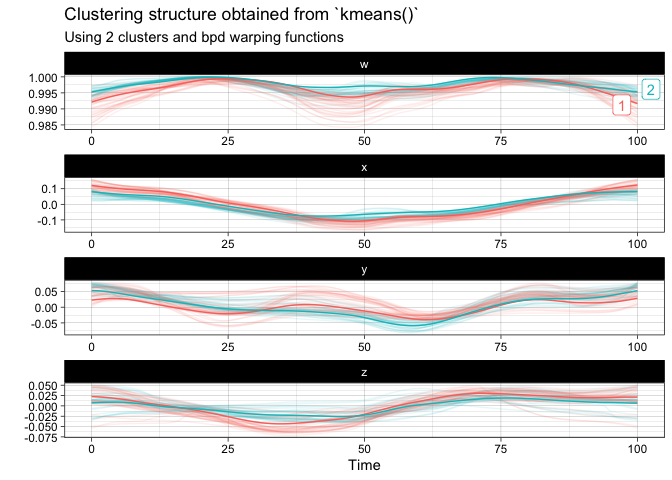The goal of squat is to provide extensions of common statistical methods for the analysis of unit quaternion time series. Available statistical methods for QTS samples are currently:

## Installation

You can install the official version from CRAN via:

install.packages("squat")

or you can opt to install the development version from GitHub with:

# install.packages("devtools")
devtools::install_github("LMJL-Alea/squat")

## Example

library(squat)

First, let us visualize the sample of QTS from the vespa64 dataset included in the package. The package provides two ways of doing this: either via a static plot or via an animated one (which uses gganimate behind the scences and will prompt you to install it in case you have not already).

Here is the static version:

plot(vespa64$igp)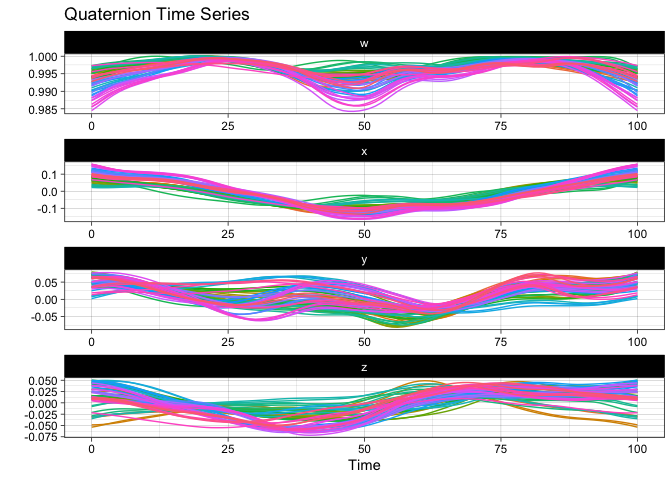You can also use ggplot2::autoplot() instead of plot() to save the resulting ggplot object for further customization. Here is the animated version: p <- ggplot2::autoplot(vespa64$igp, with_animation = TRUE)
gganimate::anim_save("man/figures/README-animated-plot.gif", p)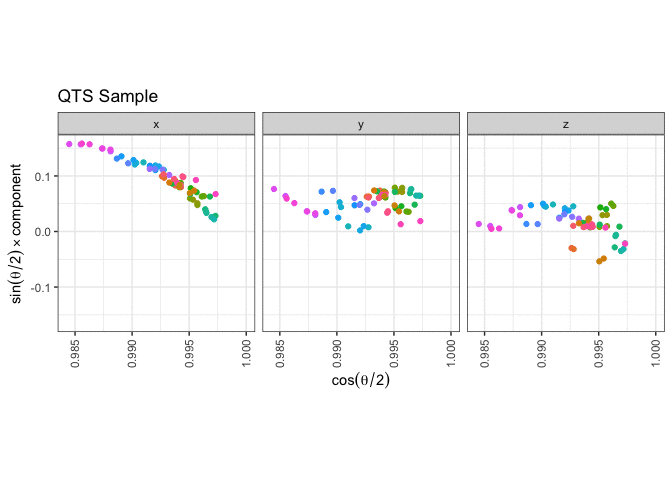You can compute the geometric mean of the sample and append it to the sample for visualization:

m <- mean(vespa64$igp) sample_and_mean <- append(vespa64$igp, m)
plot(sample_and_mean, highlighted = c(rep(FALSE, 64), TRUE))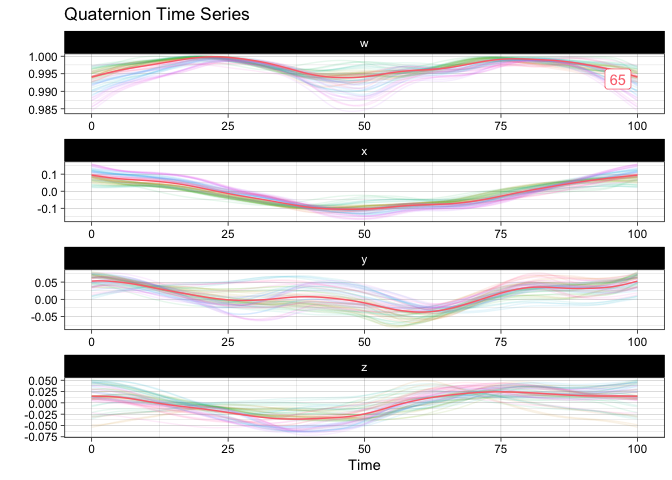You can compute the pairwise distance matrix (based on the DTW for now):

D <- distDTW(vespa64$igp) C <- exp(-D / (sqrt(2) * 4 * bw.SJ(D))) |> as.matrix() |> corrr::as_cordf() corrr::network_plot(C) #> Warning: ggrepel: 1 unlabeled data points (too many overlaps). Consider #> increasing max.overlaps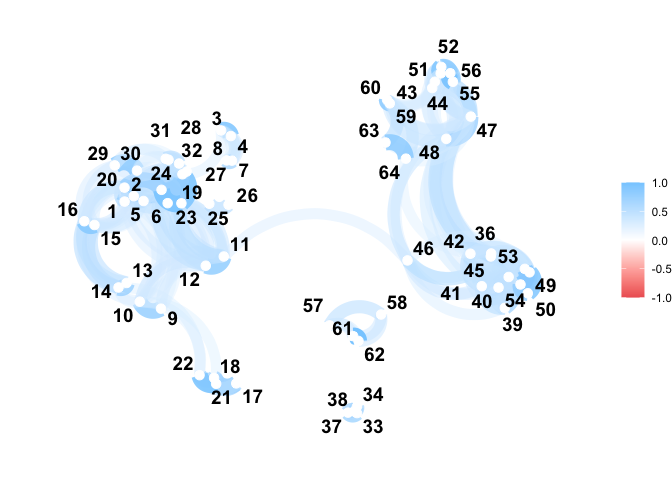You can perform tangent principal component analysis and visualize it: tpca <- prcomp(vespa64$igp)
plot(tpca, what = "PC1")
#> The original_space boolean argument is not specified. Defaulting to TRUE.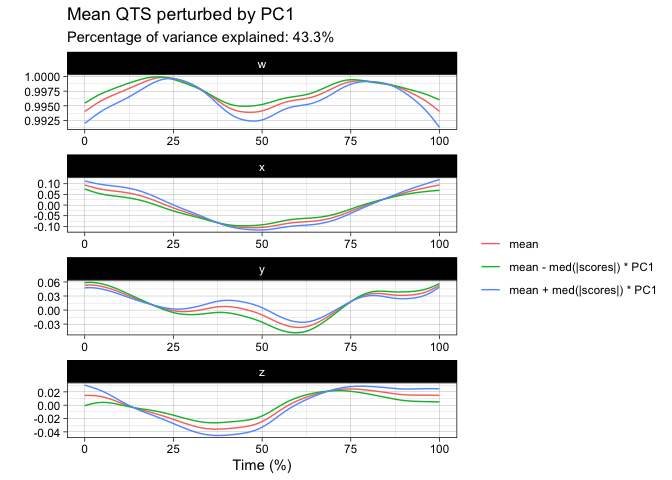plot(tpca, what = "scores")
#> The plane length-2 integer vector argument is not specified. Defaulting to
#> 1:2.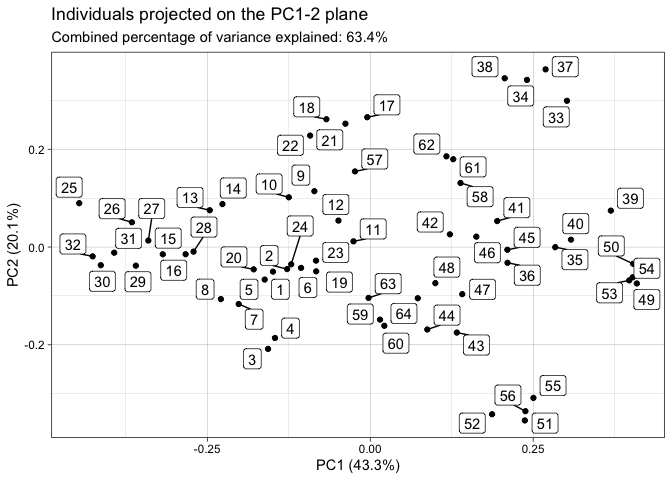screeplot(tpca)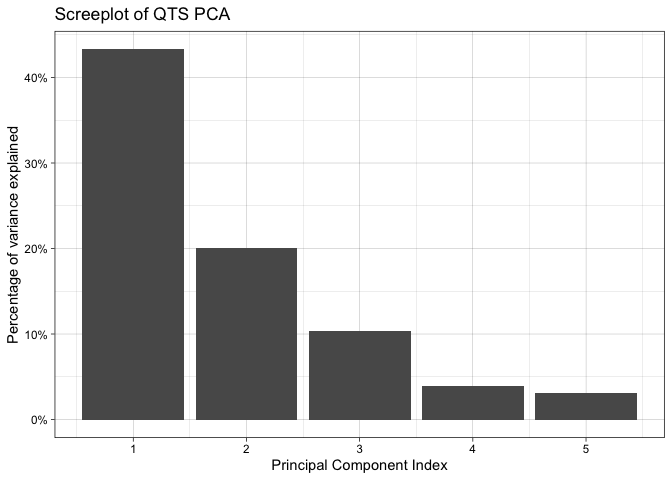You can finally perform a k-means clustering and visualize it:

km <- kmeans(vespa64\$igp, k = 2)
plot(km)